# Odds ratios, collapsibility, marginal vs. conditional, GEE vs GLMMs

Generalised estimating equations (GEEs) and generalised linear mixed models (GLMMs) are two approaches to modelling clustered or longitudinal categorical outcomes. Here I will focus on the common setting of a binary outcome. As is commonly described, the two approaches estimate different effect measures, with GEEs targeting so called marginal effects, and GLMMs targeting conditional or subject specific effects. Understanding the difference between these is potentially quite tricky I think.

Single time point
Consider a simple setting of a randomised trial with two treatments, where we observe a binary outcome Y. Let Z denote whether the patient was randomised to active (Z=1) or control (Z=0) treatment. A simple analysis of this trial would be to examine how the distribution of Y (i.e. the probability that Y=1) differs depending on whether the patient was randomised to Z=1 or Z=0. One approach to this would be to fit a logistic regression model, where Y is the outcome and Z is the sole covariate: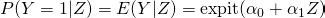where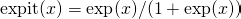.

Of course we would rarely believe that each patient’s outcome only depends on which treatment they receive. There will always be other factors which influence the probability that Y=1. This doesn’t mean the previous model was wrong in any way – it simply empirically describes how the distribution of Y depends on Z. It compares the odds of Y=1 between a random patient assigned Z=1 with the odds of Y=1 for another random patient assigned Z=0. It doesn’t imply that the odds of Y=1 increase by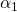for each particular patient were they to receive active instead of control treatment.

For the sake of illustration, suppose there is another factor, X, which is binary, and which influences the probability that Y=1. Suppose that a correct model for Y given Z and X is again a logistic regression: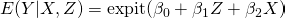Now, even though X and Z are (population) independent by virtue of randomisation, so there is no confounding, it turns out that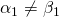. This is because, by the law of total expectation: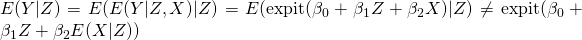For other so called link functions, such as the identity (linear regression) or the log link, these two quantities are equal, and so the effects are so called collapsible. Unfortunately with logistic regression, it doesn’t hold. If however the effect of X is small, so that the variance of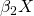is small, the model is approximately collapsible by using the delta method. In this case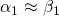. As the effect of X gets larger, the bigger the difference between the parameters.

So far we have been talking about true population parameters. The lack of collapsibility for the odds ratio also occurs with the sample estimates, even when X and Z are exactly ‘sample independent’. To illustrate in R:

> set.seed(1234)
> n <- 100
> x <- c(rep(0,n/2),rep(1,n/2))
> z <- rep(c(rep(0,n/4),rep(1,n/4)),2)
> table(x,z)
z
x    0  1
0 25 25
1 25 25
>
> expit <- function(x) {
+   exp(x)/(1+exp(x))
+ }
> pr <- expit(-1+2*x+z)
> y <- 1*(runif(n)
> summary(glm(y~z, family="binomial"))

Call:
glm(formula = y ~ z, family = "binomial")

Deviance Residuals:
Min       1Q   Median       3Q      Max
-1.4294  -1.3172   0.9448   1.0438   1.0438

Coefficients:
Estimate Std. Error z value Pr(>|z|)
(Intercept)   0.3228     0.2865   1.126    0.260
z             0.2526     0.4110   0.615    0.539

(Dispersion parameter for binomial family taken to be 1)

Null deviance: 133.75  on 99  degrees of freedom
Residual deviance: 133.37  on 98  degrees of freedom
AIC: 137.37

Number of Fisher Scoring iterations: 4

> summary(glm(y~z+x, family="binomial"))

Call:
glm(formula = y ~ z + x, family = "binomial")

Deviance Residuals:
Min       1Q   Median       3Q      Max
-1.9237  -0.9494   0.5848   0.6733   1.4240

Coefficients:
Estimate Std. Error z value Pr(>|z|)
(Intercept)  -0.5632     0.3731  -1.509    0.131
z             0.3106     0.4564   0.680    0.496
x             1.9319     0.4698   4.113 3.91e-05 ***
---
Signif. codes:  0 ‘***’ 0.001 ‘**’ 0.01 ‘*’ 0.05 ‘.’ 0.1 ‘ ’ 1

(Dispersion parameter for binomial family taken to be 1)

Null deviance: 133.75  on 99  degrees of freedom
Residual deviance: 113.98  on 97  degrees of freedom
AIC: 119.98

Number of Fisher Scoring iterations: 4


Here we see that the log odds ratio for the effect of z increasing by 1 is 0.2526 when we don’t adjust for x, and increases to 0.3106 when we do adjust for x. This change in coefficient occurs despite the fact that x and z are perfectly sample independent/balanced.

For another exposition on the lack of collapsibility for the odds ratio, I’d recommend looking at fine point 4.3 in part 1 of Hernan and Robin’s upcoming book on causal inference. As they state:

Now consider a hypothetical effect measure (other than the risk ratio or the risk difference) such that the population effect measure were not a weighted average of the stratum-specific measures. That is, the population effect measure would not necessarily lie inside of the range of values of the stratum-specific effect measures. Such effect measure would be an odd one. The odds ratio (pun intended) is such an effect measure, as we now discuss.

I entirely agree, and remember being thoroughly confused when the lack of collapsibility was first taught to me.

In practice we may collect a series of baseline covariates X, and adjust for them in our analysis. In this case, the estimated odds ratio for the effect of Z is a conditional odds ratio, conditional on the covariates X we have adjusted for. No doubt there are other characteristics of the patients which, if we had measured them, we could adjust for, and we could then report odds ratios conditional on these as well. These conditional odds ratios for the treatment effect would be larger than the odds ratios we estimate when we don’t adjust for these effects. However, without additional information, with a single time point there is no way to incorporate or adjust for these unobserved covariates. The situation however changes once we move to a clustered or longitudinal setting…

Clustered/longitudinal data
Consider the longitudinal setting, where each patient has their binary outcome measures at a series of follow-up visits. In the GEE approach, we can model how the probability that Y=1 changes over time, and how it differs between the two treatment groups. This is akin to the single time point setting where we simply included Z as the only covariate, but is complicated by the repeated measurements. A very simple model might assume that the odds of the outcome being 1 did not vary by visit, but only depended on a patient’s randomised treatment. Alternatively we might adjust for some baseline covariates, analogous to the single time point setting. The GEE approach is said to be marginal, in the sense that is marginal with respect to omitted patient level covariates/effects which are not included as covariates.

We said before that with a single time point we could not hope to adjust for unmeasured patient level covariates which we may hypothesize impact on the probability that a patient’s outcome is 1. With clustered or longitudinal data, we now have the opportunity to. The reason why this is possible is because of the fact we have repeated outcomes measured on the same patient (or cluster). Because of this, we are able to get a handle on the magnitude of any patient level unmeasured covariate effects, or patient level heterogeneity. Let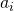denote a patient level random effect with mean zero and some distribution, which acts additively on the log odds scale. Suppose that there are very strong unmeasured patient level effects – this would correspond to the variance ofbeing large. For a patient with a high value of, their odds of Y=1 would tend to be larger at all time points. This would manifest itself in the patient’s outcomes tending to very often (across time points) being 1. Conversely, a patient with a lowwould tend to usually have outcome 0. This means the within-patient variation in outcome is low, or put another, the correlation between two outcomes measured on the same patient is high. A generalised mixed effects logistic regression model can include a term for the random effect, and with distributional assumptions, estimate their variance. If instead the within patient variation in outcomes was larger, this would indicate that the variance ofis smaller.

The GLMM approach adjusts or conditions on these patient level random effects. As such, the odds ratio (or odds ratios, depending on the model specification) for the effect of treatment are conditional on the. We can viewas simply another covariate, and thus the treatment effect odds ratio conditional onwill be larger than the one being estimated by the GEE, which does not condition on.

Which sort of odds ratio (if an odds ratio), and hence modelling approach, is more appropriate to estimate? The GLMM approach (subject specific or conditional approach) attempts to get closer to the effect of treatment for individual patients, and so some have argued this is more relevant for clinicians and patients. Conversely, the population average or marginal effects are argued to be more relevant for policy or population level decisions. One of the most extensive treatments of this question is the chapter “Targets of Inference in Hierarchical Models” by Stephen Raudenbush in the CRC Press Longitudinal Data Analysis Handbook, which I would recommend for further reading. This chapter obviously goes into much more detail than I have here, and in particular focuses on the other potential aspects that might be of interest, for example quantifying the amount of between patient heterogeneity in trajectories over time. The introductory chapter “Parametric modeling of longitudinal data: introduction and overview” by Fitzmaurice and Verbeke of the same book also contains a very nice description of the preceding issues.

I recall seeing a relatively recent paper discussing the choice in the particular context of randomised trials, but now cannot find it. If anyone has relevant papers on the topic, please add the details in a comment.

### 1 thought on “Odds ratios, collapsibility, marginal vs. conditional, GEE vs GLMMs”

This site uses Akismet to reduce spam. Learn how your comment data is processed.